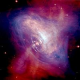# Four leading candidates for ‘A Theory of Everything’

Theory of Everything (ToE) is a hypothetical theory that completely explains and links together all physical aspects of the universe. Such a theory would merge the four known fundamental forces of the universe together. The Grand Unified Theory (GUT) successfully unites three of the four fundamental forces, namely the strong nuclear force, the weak nuclear force and the electromagnetic force. The idea is to marry these three forces (merged as one in GUT) to gravity (presently described using general relativity).

Some physicists believe that they are on the verge of finding a Theory of Everything. In this article, we present four leading candidates for a Theory of Everything. It is possible that one of these theories might become the ultimate theory of the universe one day.

### 1. String Theory

String Theory is the most popular ToE presently. According to string theory, at the most basic level, everything in our universe is made up of tiny identical vibrating strings. Different strings vibrate at different frequencies to generate different fundamental particles that make our universe. String theory also proposes a particle named as ‘graviton’ that carries the force of gravity.

The problem with string theory is that it requires additional number of dimensions for its consistency. It proposes 9 spatial + 1 temporal dimensions for our universe. Also, the strings in string theory are very tiny (of the order of 10⁻³⁵ meters or 10²⁰ times smaller than a proton). Hence, detecting these strings directly using current technology is impossible.

### 2. Loop Quantum Gravity

Loop Quantum Gravity or LQG is another ToE which is popular among the physicists. Albert Einstein taught us that space and time are joined in one entity: space-time. But LQG treats both space and time as discrete quantities. According to the loop quantum gravity theory, space is not continuous but is instead broken up into tiny quantas which are 10⁻³⁵ meters across. These quantas of space are then connected by links to form the space we experience.

LQG predicts that Big Bang was not the beginning of our universe. Instead, our universe was formed after the collapse of a previous universe in an event known as the Big Bounce. Applying LQG to a black hole eliminates the need for a ‘singularity’. LQG predicts the existence of a portal to another universe instead of a singularity at the center of a black hole.

Although LQG makes some interesting predictions, it goes against the basic idea of Einstein’s theory of relativity. Theory of relativity has passed many rigid tests in the recent years (For example: the detection of gravitational waves). Therefore, space and time might not be discrete as predicted by LQG.

### 3. Casual dynamical triangulation (CDT)

CDT might look similar to LQG but it is not. CDT divides the spacetime into tiny triangular sections (simplex) known as pentachronons. These triangular sections are then glued together in a variety of ways to create curved spacetimes. CDT models the structure of space time both at the planck scale and the cosmic scale making it a promising candidate for a ToE.

### 4. E8 (An Exceptionally Simple Theory of Everything)E8 is 248 dimensional mathematical object (Image: Cjean42/Wikimedia Commons,CC BY-SA 4.0)

According to the E8 theory, a 248 dimensional mathematical object is a thoery of everything. In the E8 theory, each elementary particle is assigned eight quantum numbers depending on the charge it has. Then, each distinct particle is placed at each of the 248 points using the eight numbers (based on their charges) as coordinates.

The E8 theory suggests that there are more elementary particles in our universe which are yet to be observed. Detecting these particles might suggest that an eight dimensional object with 248 segments might be a theory of everything.

1.raunaksarada123 says: